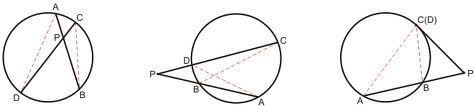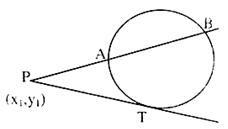×#### Thank you for registering.

One of our academic counsellors will contact you within 1 working day.

Click to Chat

1800-1023-196

+91-120-4616500

CART 0

• 0

MY CART (5)

Use Coupon: CART20 and get 20% off on all online Study Material

ITEM
DETAILS
MRP
DISCOUNT
FINAL PRICE
Total Price: Rs.

There are no items in this cart.
Continue Shopping```Radical Axis and Power of a Point with respect to a Circle

Table of Content

Power of a Point with Respect to a Circle

Related Resources

Power of a Point with Respect to a CircleIf we have a point (say) P and a circle, then  we draw two lines passing through the point P and intersecting the circle in points A and B, C and D. Then the following holds true:

AP. BP = CP. DP

Now, we can have various cases according to whether the point P lies inside or outside the circle.The various possible cases are:

Both the lines are secants and intersect inside the circle.

One line is a secant and the other is a tangent.

Both the lines are secants but they intersect outside the circle.Now, we discuss these three cases in brief:

Case 1: The point P lies inside the circle and hence in this case we have AP.BP = CP.DP

This is also called as the theorem of intersecting chords,

Case 2: We have two secants which intersect at point P lying outside the circle.

In this case, we have PB.PA = PD.PC

This is also known as the theorem of intersecting secants.

Case 3: The power of a point P(x1, y1) with respect to a given circle defined asPower = PA × PB

Where A and B are the points on the circle where the line PAB intersects it. (See figure given above).

Clearly when A and B coincide to T then we have

Power = PT2 = Square of the length of tangent from point P(x1, y1) to the circle.

Note that the power of a point is negative if the point is inside the circle.

This radical axis of two circles is the locus of a point from which the tangent segments to the two circles are of equal lengths.

In general S – S’ = 0 represents the equation of the Radical Axis to the two circles

i.e. 2x(g – g’) + 2y(f – f’) + c – c’ = 0

where S ≡ x2 + y2 + 2gx + 2fy + c = 0

and S’ ≡ x2 + y2 + 2g’x + 2f’y + c’ = 0

(i) If S = 0 and S’ = 0 intersect in real and distinct points then S – S’ = 0 is the equation of the common chord of the two circles.

(ii) If S’ = 0 and S = 0 touch each other, then S – S’ = 0 is the equation of the common tangent to the two circles at the point of contact.The radical axis of two circles is perpendicular to the line joining the centres.

The radical axis passes through the mid-point of the line joining the centres of the two circles provided the radii of the two circles are equal.

The radical axis of two circles bisects their direct common tangents.

The radical axis of the two circles which intersect each other is the extension of their common chord.

A system of circles, every two of which have the same radical axis is called a coaxial system.

A coaxial system of circles have one point on each side of the radical axis. These points are also termed to be the limiting points of the radical axis.

All the circles which pass through these limiting points are orthogonal to the coaxial system.

The pair of circles which don’t have a radical axis are cocentric.

If two circles cut a third circle orthogonally, then the radical axis of the two circles will pass through the centre of the third circle.

The radical axis of three circles taken two at a time are concurrent and the point of concurrency is known as the radical centre.

If we have a triangle ABC, and there are three circles having diameters as AB, BC and CA respectively, then the radical centre of the three circles is also the orthocentre of the triangle ABC.

Result:

The radical axis of three circles, taken in pairs are concurrent.

Proof:

Let the equations of the three circles be

S1 = x2 + y2 + 2g1x + 2f1y + c1 = 0

S2 = x2 + y2 + 2g2x + 2f2y + c2 = 0

S3 = x2 + y2 + 2g3x + 2f3y + c3 = 0  …..... (1)

Now, the radical axis of S1 is obtained by subtracting the equations of these circles. Hence, it is

S1 – S2 = 0             …... (2)

Similarly, the radical axis of S2 and S3 is S2 – S3 = 0             …... (3)

The lines (2) and (3) meet at a point whose coordinates (X, Y) satisfy S1 – S2 = 0 and S2 – S3 = 0.

So, the coordinates (X, Y) satisfy (S1 – S2) + (S2 – S3) = 0.

Hence, (X, Y) satisfy S1 – S3 = 0  …...(4)

But, this equation is the radical axis of the circles S1 and S3 and hence the three radical axes are concurrent.

Illustration:

Prove that the circle x2 + y2 – 6x – 4y + 9 = 0 bisects the circumference of the circle x2 + y2 – 8x – 6y + 23 = 0

Solution:

The given circles are S1= x2 + y2 – 6x – 4y + 9 = 0

S2 = x2 + y2 – 8x – 6y + 23 = 0

Equation of the common chord of circles (1) and (2) which is also the radical axis of the circles S1 and S2 is

S1 – S2 = 0. or 2x + 2y -14 = 0

This gives x + y – 7 = 0

Now, the centre of the circle S2 is (4, 3). Also, Clearly, line (3) passes through the point (4, 3) and hence line (3) is the equation of the diameter of the circle (2). Hence, circle (1) bisects the circumference of circle (2).

Related Resources

For getting an idea of the type of questions asked, refer the previous year papers.

Fast Track your revision with the ultimate Revision Notes on Circle.

To read more, Buy study materials of Circles comprising study notes, revision notes, video lectures, previous year solved questions etc. Also browse for more study materials on Mathematics here.
```### Course Features

• 731 Video Lectures
• Revision Notes
• Previous Year Papers
• Mind Map
• Study Planner
• NCERT Solutions
• Discussion Forum
• Test paper with Video Solution